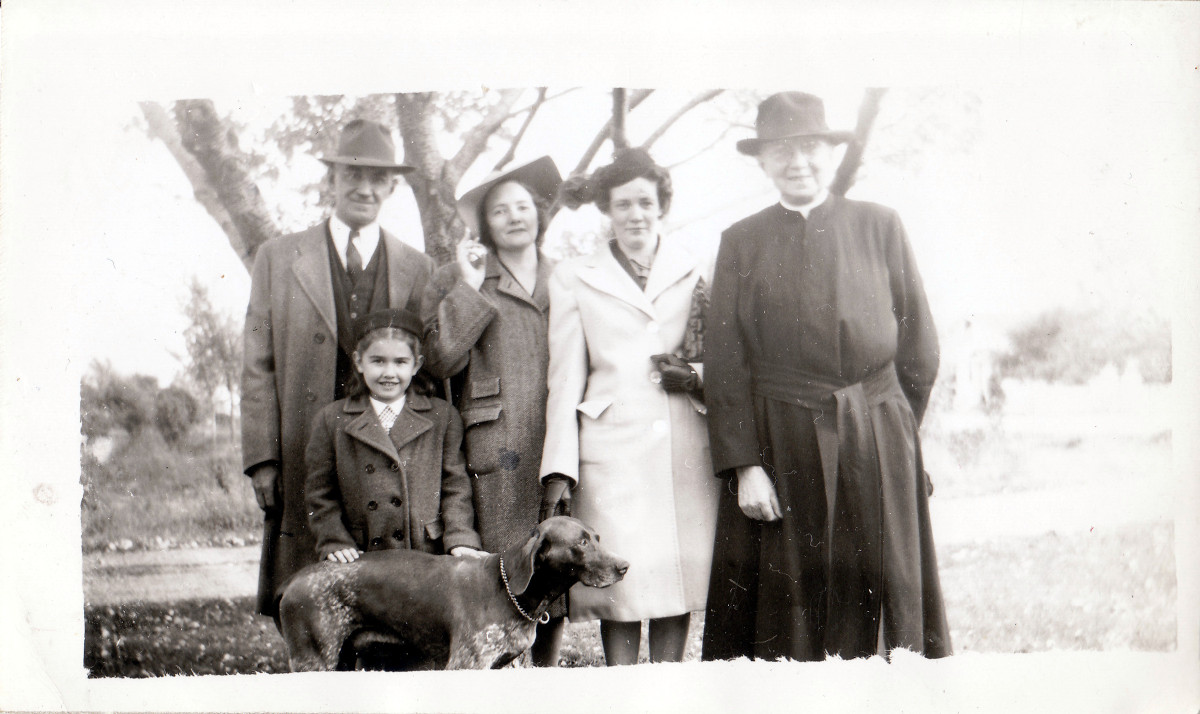## Sunday Outing

Place mouse over face for identification of personDescription Sunday Outing Date / Time 1947-00-00 Country / State / County / City / Placename xxx Persons (t-b,l-r) Noah Louis Goulah, Nannette Marie Goulah, Florence Agnes Goulah, UNK, Father Kinlin
```lib card:
dc:
title 		=	Sunday Outing
creator 	=	Jerome Goulet
subject 	=
description 	=	Original (scanned analog or digital), names to faces, stored to LibCard "format", within image exif:
publisher 	=	Jerome Goulet
contributor 	=
date 		=	1947-00-00
resourcetype 	=
iformat 	=
if_mime 	=	image/jpeg
if_resolution 	=	600x600
if_dimensions 	=
if_dim_x 	=	1200
if_dim_y 	=	714
resourceID 	=
source 		=	photograph (analog or digital)
language 	=	eng
lc:
when:
dateUTC		=	1947-00-00
timeUTC		=	0000
tz		=	+0000

dateLocal	=	1947-00-00
timeLocal	=	0000
where:
pName		=	x
lat		=	x
lon		=	x
who:
person:
name:
surName		=	Goulah
givenName	=	Noah Louis
aka		=	NLG1893
relation	=
pip:	(person in picture)
boxX		=	337
boxY		=	170
boxWidth	=	64
boxHeight	=	64
label		=
uri		=
person:
name:
surName		=	Goulah
givenName	=	Nannette Marie
aka		=	NMG1937
relation	=
pip:	(person in picture)
boxX		=	357
boxY		=	336
boxWidth	=	64
boxHeight	=	64
label		=
uri		=
person:
name:
surName		=	Goulah
givenName	=	Florence Agnes
aka		=	FAG1930
relation	=
pip:	(person in picture)
boxX		=	484
boxY		=	185
boxWidth	=	61
boxHeight	=	61
label		=
uri		=
person:
name:
surName		=	UNK
givenName	=
aka		=
relation	=
pip:	(person in picture)
boxX		=	613
boxY		=	200
boxWidth	=	64
boxHeight	=	64
label		=
uri		=
person:
name:
surName		=	Kinlin
givenName	=	Father
aka		=	FrK
relation	=
pip:	(person in picture)
boxX		=	755
boxY		=	116
boxWidth	=	82
boxHeight	=	82
label		=
uri		=
what:
eventName	=	Sunday Outing
```